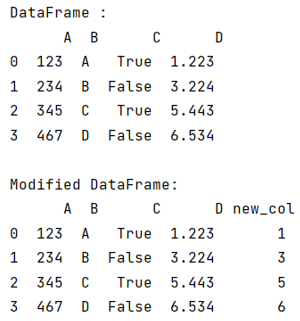# Python Pandas - Get first letter of a string from column

Given a Pandas DataFrame, we have to get the first letter of a string from column.
Submitted by Pranit Sharma, on August 04, 2022

Pandas is a special tool that allows us to perform complex manipulations of data effectively and efficiently. Inside pandas, we mostly deal with a dataset in the form of DataFrame. DataFrames are 2-dimensional data structures in pandas. DataFrames consist of rows, columns, and data.

Sometimes, we work we want to gain some insights from the values of each column, for this we might need to study each letter of strings from columns.

To get the first letter of a string from the column, we will first create a Dataframe with multiple columns, we will then create a new column in this dataframe and assign the first letter of value of the first column to it

Let us understand with the help of an example,

## Python code to get first letter of a string from column

```# Importing pandas package
import pandas as pd

# Creating a dictionary
d = {
'A':[123,234,345,467],
'B':['A','B','C','D'],
'C':[True,False,True,False],
'D':[1.223,3.224,5.443,6.534]
}

# Creating a dataframe
df = pd.DataFrame(d)

# Display Dataframe
print("DataFrame :\n",df,"\n")

# Making a new column
df['new_col'] = df['D'].astype(str).str

# Display modified DataFrame
print("Modified DataFrame:\n",df)
```

Output:Languages: » C » C++ » C++ STL » Java » Data Structure » C#.Net » Android » Kotlin » SQL
Web Technologies: » PHP » Python » JavaScript » CSS » Ajax » Node.js » Web programming/HTML
Solved programs: » C » C++ » DS » Java » C#
Aptitude que. & ans.: » C » C++ » Java » DBMS
Interview que. & ans.: » C » Embedded C » Java » SEO » HR
CS Subjects: » CS Basics » O.S. » Networks » DBMS » Embedded Systems » Cloud Computing
» Machine learning » CS Organizations » Linux » DOS
More: » Articles » Puzzles » News/Updates